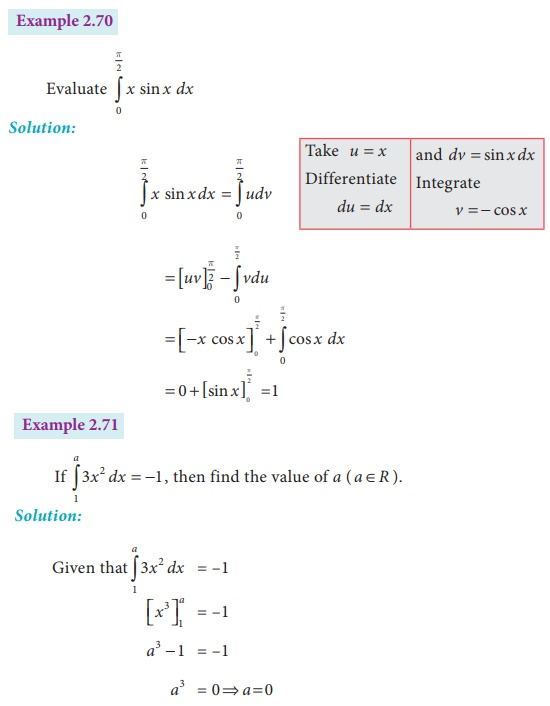Home | | Business Maths 12th Std | The fundamental theorems of Integral Calculus: Exercise and Example Solved Problems

# The fundamental theorems of Integral Calculus: Exercise and Example Solved Problems

Maths: Integral Calculus: Definite integrals: The fundamental theorems of Integral Calculus: Exercise and Example Solved Problems with Answer, SolutionExercise 2.8

I Using second fundamental theorem, evaluate the following:II Evaluate the following:Tags : Definite integrals , 12th Business Maths and Statistics : Chapter 2 : Integral Calculus - I
Study Material, Lecturing Notes, Assignment, Reference, Wiki description explanation, brief detail
12th Business Maths and Statistics : Chapter 2 : Integral Calculus - I : The fundamental theorems of Integral Calculus: Exercise and Example Solved Problems | Definite integrals